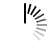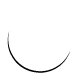HTML area alt Attribute

```<!DOCTYPE html>
<html>
<body>
<h2>Learning</h2>
<p>Learn these technologies with ease....</p>
<img src = /images/usemap.gif alt = "usemap" border = "0" usemap = "#tutorials"/>
<map name = "tutorials">
<area shape = "poly" coords = "74,0,113,29,98,72,52,72,38,27"
href = "/perl/index.htm" alt = "Perl Tutorial" target = "_blank" />
<area shape = "rect" coords = "22,83,126,125" alt = "HTML Tutorial"
href = "/html/index.htm" target = "_blank" />
<area shape = "circle" coords = "73,168,32" alt = "PHP Tutorial"
href = "/php/index.htm" target = "_blank" />
</map>
</body>
</html>```